﻿ 公开市场操作、利率走廊与市场拆借利率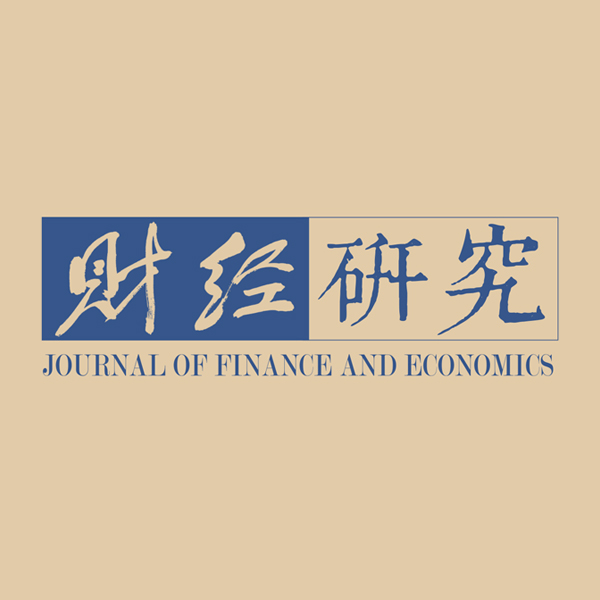《财经研究》
2019第45卷第7期

Open Market Operations, Interest Rate Corridors and the Inter-bank Offered Rate
Huang Zhigang, Liu GuofangSchool of Finance, Central University of Finance and Economics, Beijing 100081, China
Key words: open market operations    interest rate corridors    inter-bank offered rate

$t = 0,1,2$
，其中
$t = 1$

$t = 2$

$[0,1]$

$\alpha$
，投资短期资产的比例为
$1 - \alpha$

$t = 0$

$t = 1$

$\mathop d\nolimits_1$
；若在
$t = 2$

$\mathop d\nolimits_2$
。假设银行
$i$
$\mathop \lambda \nolimits_i$

$t = 1$

$\mathop \lambda \nolimits_i \mathop d\nolimits_1$

$\mathop \lambda \nolimits_i$

$\mathop \lambda \nolimits_i \in [0,1]$
，其密度函数为
$f\left( {\mathop \lambda \nolimits_i } \right)$
$E\left( {\mathop \lambda \nolimits_i } \right) = 0.5$
。与已有文献不同，本文假设流动性冲击是异质性的，这样就可以刻画银行的流动性异质特征和差异化的流动性需求。

$t = 1$

${z_m}$
，但存在流动性调整成本，设为出售资产规模的函数：
${c_i} = 0.5\phi A_i^2$
，其中
${A_i}$

$t = 1$

$t = 0$

1999年以来，公开市场操作已成为我国央行货币政策日常操作的重要工具。人民银行利用该工具吞吐流动性。与欧美国家不同，我国的公开市场操作具有数量型中介目标（戴根有，2003；伍戈和李斌，2016）。为了刻画这一特点，假设央行通过公开市场向每家银行注入流动性

$\eta$
，则向整个市场投放的流动性为
$m = \int \eta di$

（一）同业拆借市场中银行的决策。在

$t = 1$

$i$

$k$

$({\lambda _i} + {\lambda _k}){d_1} > 2(1 - \alpha + \eta )$
。管理流动性时，双方会在出售长期资产和同业拆借之间进行权衡。银行
$i$

 ${\Pi _i} = R(\alpha - {A_i}) - 0.5\phi A_i^2 - (1 + {r_{ik}}){B_i} - (1 - {\lambda _i}){d_2}$ (1)

${A_i}$

$i$

${A_i} > 0$
${B_i}$

$i$

${B_i} = {\lambda _i}{d_1} - (1 - \alpha ) - {z_m}{A_i} - \eta$
。该式表明，银行
$i$

${B_i} < 0$

${\lambda _i}{d_1}$

$1 - \alpha$
、出售长期资产获得的流动性
${z_m}{A_i}$

$\eta$
${r_{ik}}$

$i$

$k$

 $1 + {r_{ik}} = \frac{R}{{{z_m}}} + \frac{\phi }{{z_m^2}}(D - 1 + \alpha - \eta )$ (2)

$D = 0.5({\lambda _i} + {\lambda _k}){d_1}$
，表示两家相遇银行的平均流动性冲击水平（简称流动性冲击水平）。

$i$

$k$

$D \in [0,1 - \alpha + \eta ]$
）。他们的整体流动性剩余较多，交易的机会成本为
$R/{z_m}$
。此时，银行
$i$

 ${\Pi '_i} = R\alpha + 1 - \alpha + \eta - {\lambda _i}{d_1} - (1 - {\lambda _i}){d_2}$ (3)

（二）银行资产配置决策。在

$t = 0$

$i$

 $\mathop {\rm{Max}}\limits_\alpha \int_{D \in [1 - \alpha + \eta ,{d_1}]} {{\Pi _i}dF\left( D \right)} + \int_{D \in [0,1 - \alpha + \eta ]} {{\Pi '_i}dF\left( D \right)} - 0.5\psi {(\alpha - {\alpha _0})^2}$ (4)

$i$

$i$

$0.5\psi {(\alpha - {\alpha _0})^2}$
${\alpha _0}$

$\psi$

$i$

$\alpha$

 $\frac{{\partial \alpha }}{{\partial \eta }} = 1 - \frac{\psi }{{\psi + \displaystyle\frac{\phi }{{z_m^2}}\tilde X + \frac{\phi }{{z_m^2}}\tilde Y + \frac{2}{{{d_1}}}(R - 1)\tilde Z}}$ (5)

$\tilde X$
$\tilde Y$
$\tilde Z$

$f\left( {\mathop \lambda \nolimits_i } \right)$
$f\left( {\mathop \lambda \nolimits_j } \right)$

${{\partial \alpha }/{\partial \eta }} \in [0,1]$
。式（5）表明，银行的长期资产投资与央行的公开市场操作正相关（
$\partial \alpha /\partial \eta > 0$
）。这意味着央行的流动性投放会减弱银行持有流动性资产的动机，从而增加银行的长期资产投资。产生这种效果的原因是，央行的公开市场操作是预期到的。在预期到的公开市场操作下，央行增加流动性时，银行会用央行提供的流动性来替代自己的流动性，本文将这种特征称为“流动性替代效应”。

（三）公开市场操作与同业拆借利率。综合以上分析，成交利率为：

 1 + {r_{ik}} = \left\{ \begin{aligned} & \frac{R}{{{z_m}}} + \frac{\phi }{{z_m^2}}[D - 1 + \alpha - \eta ]{\rm{ }} & 1 - \alpha + \eta \leqslant D \leqslant {d_1} \\ & \frac{R}{{{z_m}}} & 0 \leqslant D \leqslant 1 - \alpha + \eta \; \\ \end{aligned} \right. (6)

$\ell$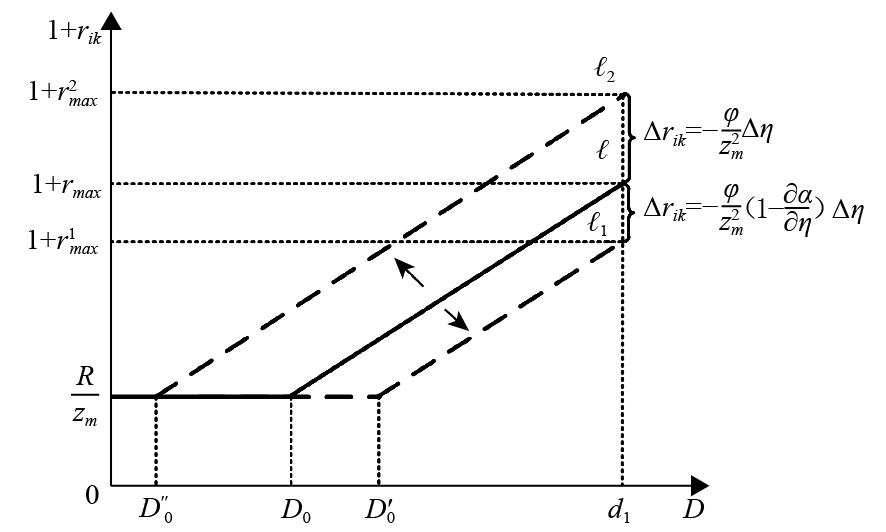图 1 公开市场操作对同业拆借利率的影响

${\eta _1}$
，银行在第0期的预期为
${\eta _0}$
。那么，
${\eta _0}$

${\eta _1} - {\eta _0}$

1. 预期到的公开市场操作。假设为了降低拆借利率水平和利率波动，央行通过公开市场增加流动性投放，且银行预期到了这一操作。此时，

$\Delta \eta {\rm{ = }}{\eta '_0} - {\eta _0} > 0$
，曲线
$\ell$

$\ell \to {\ell _{\rm{1}}}$
），成交利率下降，
$\Delta {r_{ik}} = - {\phi /{z_m^2}}(1 - {{\partial \alpha }/{\partial \eta }})\Delta \eta$
。因此，拆借利率下降，其分布区间收窄，从
$[{R/{{z_m}}},1 + {r_{max}}]$

$[{R/{{z_m}}},1 + r_{max}^1]$

2. 未预期到的公开市场操作。当央行实际投放的流动性低于银行的预期投放（

$\Delta \eta {\rm{ = }}{\eta _1} - {\eta _0} < 0$
）时，
$\Delta {r_{ik}} = - ({\phi /{z_m^2}})\Delta \eta > 0$
，拆借利率上升。曲线
$\ell$

$\ell \to {\ell _2}$
），拆借利率波动也大幅上升，分布区间从
$[{R/{{z_m}}},1 + {r_{max}}]$

$[{R/{{z_m}}},1 + r_{max}^2]$

3. “钱荒”的形成机制。2013年6月，在我国银行间市场发生了“钱荒”，本文的模型可以对此进行分析。美国“次贷”危机之后，为了缓解外需疲弱所带来的经济下滑，我国开始采取宽松的货币政策，在很长一段时间内，源源不断地给市场提供流动性。在这种情况下，银行预期央行会持续提供流动性，所以

${\eta _0}$

${\eta _1} < {\eta _0}$
。根据上述分析，这种未预期到的公开市场操作会带来整个银行间市场流动性的紧张。因此，当央行流动性供给枯竭时，银行自身的流动性也非常少，结果必然出现“钱荒”现象。这里的分析不同于已有文献对“钱荒”的解释，如“货币空转说”（朱孟楠和侯哲，2014；杨光和孙浦阳，2015）和“QE退出说”（董小君，2013；张明，2013；张明等，2016）。本文认为，货币在金融系统中空转和美国QE政策宣布退出等是导致银行间市场流动性减少的原因，但央行未及时采用公开市场操作来调节流动性供给、保持流动性稳定，从而引发了“钱荒”。

${r^s}$
；而贷款便利工具是指银行可以随时自由地向央行贷款，设其利率为
${r^f}$

（一）同业拆借市场中银行的决策。引入利率走廊之后，除了同业拆借和出售长期资产外，银行还可以利用利率走廊来管理流动性。利率走廊将同业拆借市场中银行的双边谈判区分为三种情况。第一，当银行

$i$

$k$

$1 + {r_{ik}} > 1 + {r^f}$
。此时，资金短缺的银行可以通过拆入流动性、出售长期资产和向央行借钱这三种方式来管理流动性，最优决策须满足三者的边际成本相等。而资金相对充裕的银行也会出售一些长期资产，使得资金的边际成本等于边际收益。此时，银行
$i$

 $\Pi _i^f = R(\alpha - {A_i}) - 0.5\phi {A_i^2} - (1 + {r^f}){B_i} - (1 - {\lambda _i}){d_2}$ (7)

$i$

$k$

 $1 + {r_{ik}} = 1 + {r^f}$ (8)

$i$

$k$

$1 + {r_{ik}} < 1 + {r^s}$
。此时，资金充裕的银行可以拆出流动性，也可以将其存入央行，同时会出售一些长期资产，使得资金的边际成本等于边际收益。而资金短缺银行的最优流动性管理决策须满足出售长期资产和同业拆借的边际成本相等。此时，银行
$i$

 $\Pi _i^s = R(\alpha - {A_i}) - 0.5\phi {A_i^2} + (1 + {r^s}){B_i} - (1 - {\lambda _i}){d_2}$ (9)

$i$

$k$

 $1 + {r_{ik}} = 1 + {r^s}$ (10)

$i$

$k$

$1 + {r^s} < 1 + {r_{ik}} < 1 + {r^f}$
。银行不使用走廊，双方根据竞价结果达成交易。银行
$i$

 ${\Pi _i} = R(\alpha - {A_i}) - 0.5\phi A_i^2 - (1 + {r_{ik}}){B_i} - (1 - {\lambda _i}){d_2}$ (11)

$i$

$k$

 $1 + {r_{ik}} = \frac{R}{{{z_m}}} + \frac{\phi }{{z_m^2}}\left( {D - 1 + \alpha } \right)$ (12)

（二）银行资产配置决策。在

$t = 0$

$i$

 $\mathop {\rm{Max}}\limits_\alpha \int_{D \in [1 - \alpha + \kappa ,{d_1}]} {\Pi _i^fdF\left( D \right)} + \int_{D \in [0,1 - \alpha + \nu ]} {\Pi _i^sdF\left( D \right)} + \int_{D \in [1 - \alpha + \nu ,1 - \alpha + \kappa ]} {{\Pi _i}dF\left( D \right)} - 0.5\psi {(\alpha - {\alpha _0})^2}$ (13)

$\alpha$

${r^f}$
${r^s}$

 $\frac{{\partial \alpha }}{{\partial {r^f}}} = - \frac{{\tilde S}}{{\psi + {{\phi \tilde V}/{z_m^2}}}},\frac{{\partial \alpha }}{{\partial {r^s}}} = - \frac{{\tilde H}}{{\psi + {{\phi \tilde V}/{z_m^2}}}}$ (14)

$\tilde S$
$\tilde V$
$\tilde H$

$f\left( {\mathop \lambda \nolimits_i } \right)$
$f\left( {\mathop \lambda \nolimits_j } \right)$

${{\partial \alpha }/{\partial {r^f}}} < 0$
${{\partial \alpha }/{\partial {r^s}}} < 0$
。式（14）表明，银行的长期资产投资与贷款便利利率负相关，即贷款便利利率越高，银行会持有越多的流动性资产、越少的长期资产。其原因在于，贷款便利利率越高，银行预期到未来面临流动性冲击时的融资成本越高，从而会投资越多的流动性资产。另外，银行的长期资产投资与存款便利利率也负相关，即存款便利利率越高，银行也会持有越多的流动性资产、越少的长期资产。其原因在于，存款便利利率越高，期望拆借利率越高，融资成本也就越高，从而银行会投资越多的流动性资产。式（14）还表明，银行的长期资产投资对存贷款便利利率变化的敏感程度与长期资产调整成本参数（
$\psi$
）负相关。

$\bar r = \left( {{r^f} + {r^s}} \right)/2$
，走廊限宽为
$\delta$
，则
${r^f} = \bar r + \delta /2$
${r^s} = \bar r - \delta /2$
。将其代入式（13），并经过上述优化分析可得：

 $\frac{{\partial \alpha }}{{\partial \delta }} = - \frac{{\tilde S - \tilde H}}{{2(\psi + {{\phi \tilde V}/{z_m^2}})}}$ (15)

${{\partial \alpha }/{\partial \delta }}$

${{\partial \alpha }/{\partial \delta }} < 0$
）；如果低流动性冲击银行较多，则后一种效应更大，限宽放宽导致银行的长期资产投资增加（
${{\partial \alpha }/{\partial \delta }} > 0$
）。这与Whitesell（2006）及Bindseil和Jabłecki（2011）的研究结论一致。但这些研究并没有指出产生这一结果的原因，本文则发现这是由银行流动性异质特征所致。

（三）利率走廊与同业拆借利率。结合式（8）、式（10）和式（12），在利率走廊背景下，同业成交利率为（计算过程留存备索）：

 1 + {r_{ik}} = \left\{ \begin{aligned} &\;1 + {r^f} \quad\quad\quad\quad\quad\quad \ \,1 - \alpha + \kappa \leqslant D \leqslant {d_1}\\ &\;\frac{R}{{{z_m}}} + \frac{\phi }{{z_m^2}}[D - 1 + \alpha ] \quad\; 1 - \alpha + \nu < {\rm{ }}D < 1 - \alpha + \kappa\\ &\; 1 + {r^s} \quad\quad\quad\quad\quad\quad \ \,\, 1 - \alpha < {\rm{ }}D \leqslant 1 - \alpha + \nu \quad \end{aligned} \right. (16)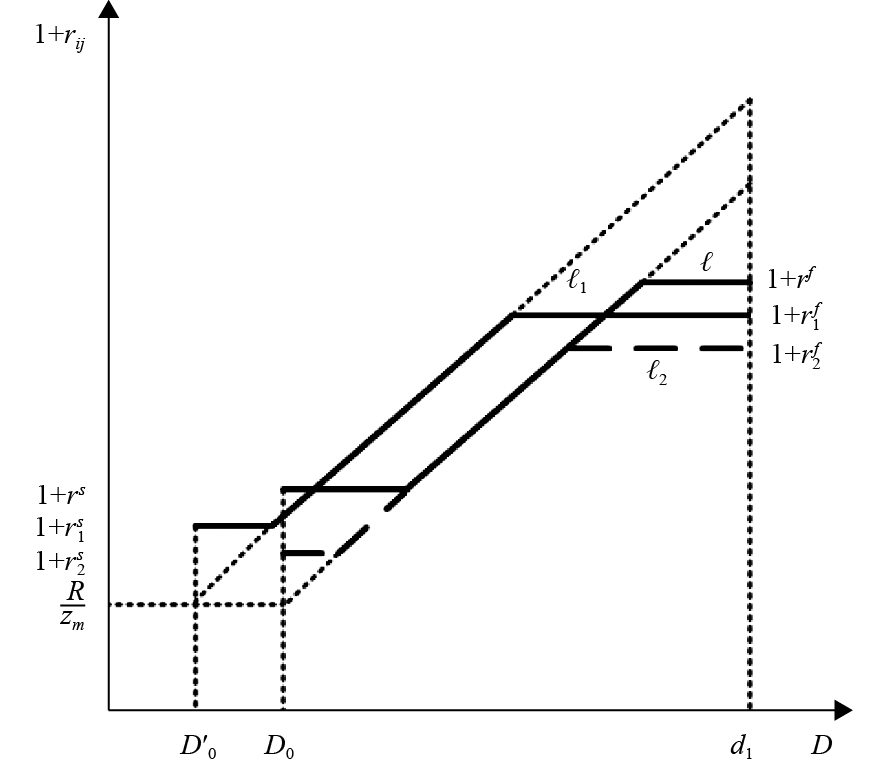图 2 利率走廊上下限对同业拆借利率的影响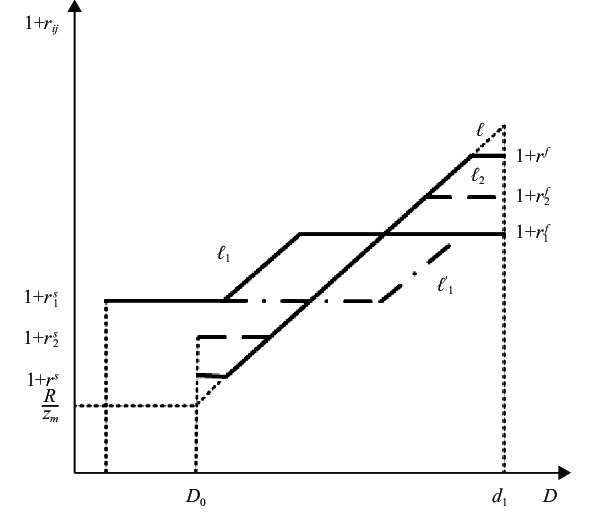图 3 利率走廊限宽对同业拆借利率的影响

1. 利率走廊上下限同幅调整对拆借利率的影响

（1）预期到的利率走廊上下限调整。假设为降低利率和维持利率波动稳定，央行同时下调走廊上下限。成交利率曲线

$\ell$

$\ell \to {\ell _1}$
），拆借利率的分布区间从
$[1 + {r^s},1 + {r^f}]$

$[1 + r_1^s,1 + r_1^f]$
，如图2所示。产生这一结果的主要原因是，在成交利率为
$1 + {r_{ik}} \in [1 + r_1^s,1 + r_1^f]$

$\eta$

$\alpha$

（2）未预期到的利率走廊上下限调整。如果走廊上下限下调是未预期到的，成交利率曲线

$\ell$

$\ell \to {\ell _2}$
），拆借利率的分布区间从
$[1 + {r^s},1 + {r^f}]$

$[1 + r_2^s,1 + r_2^f]$
，如图2所示。由于利率走廊调整未预期到，银行的资产配置维持在原来的状态，持有的流动性资产不受利率走廊调整的影响，因此不依赖利率走廊的银行的拆借利率不受影响。但有三类银行会受影响：第一类是原本就依赖利率走廊的银行。对于原来依赖贷款便利的银行（D很大），由于利率走廊下调，资金成本降低；对于原来依赖存款便利的银行（D很小），由于利率走廊下调，央行存款收益下降。第二类是原来不依赖走廊、现在依赖走廊的银行（D较大）。这些银行原来通过拆借市场融资的利率较高，贷款便利利率下调后，他们转向依赖央行融资，从而融资成本下降。第三类是原来依赖走廊、现在不依赖走廊的银行（D较小）。这些银行原来将多余的资金存在央行，收益率较高；存款便利利率下降后，他们将以高于此的利率拆出资金。

2. 利率走廊限宽调整对拆借利率的影响

（1）预期到的利率走廊限宽调整。假设为抑制利率波动和保持目标利率不变，央行决定收窄走廊的限宽。如果该政策是被预期到的，成交利率曲线

$\ell$

$\ell \to {\ell _1}$
），也可能向右平移（
$\ell \to {\ell '_1}$
），因为
${{\partial \alpha }/{\partial \delta }}$

$[1 + {r^s},1 + {r^f}]$

$[1 + r_1^s,1 + r_1^f]$
，如图3所示。

（2）未预期到的利率走廊限宽调整。如果是未预期的走廊限宽收窄，成交利率曲线

$\ell$

${\ell _2}$
）。拆借利率的分布区间从
$[1 + {r^s},1 + {r^f}]$

$[1 + r_2^s,1 + r_2^f]$
，如图3所示。由于未预期到限宽调整，银行不会调整资产配置，因此不存在“流动性替代效应”。那么，同业市场货币数量变动等于央行货币释放。此时，同业市场货币数量增加，加权平均拆借利率下降。央行较好地实现了扩张性货币政策的意图。

 ${L_i} \leqslant \rho \alpha$ (17)

${L_i}$

$i$

$\rho$

$0 \leqslant \rho \leqslant 1$
）。

（一）银行决策。在

$t = {\rm{1}}$

$i$

$k$

$i$

 $\Pi _i^{'f} = R(\alpha - {A'_i}) - 0.5\phi A_i^{'2} - (1 + {r'_{ik}}){B_i} - (1 + {r^f})\rho \alpha - (1 - {\lambda _i}){d_2}$ (18)

 $1 + {r'_{ik}} = \frac{R}{{{z_m}}} + \frac{\phi }{{z_m^2}}[D - 1 + (1 - \rho )\alpha ]$ (19)

$t = 0$

 $\frac{{\partial \alpha }}{{\partial \rho }} > 0$

$\rho$

$\rho$

（二）抵押政策与同业拆借利率。结合式（8）、式（10）、式（12）和式（19）得到成交利率（留存备索）。如图4所示，当银行从央行融资受到利率走廊抵押要求的约束时，成交利率会突破利率走廊上限。其原因在于，银行虽然有意愿从央行融资，但是没有足够的抵押品，因此只能在同业市场上拆借；同时，由于银行的流动性需求较大，而对方银行提供流动性资金的边际成本递增，因此成交利率超过走廊上限水平。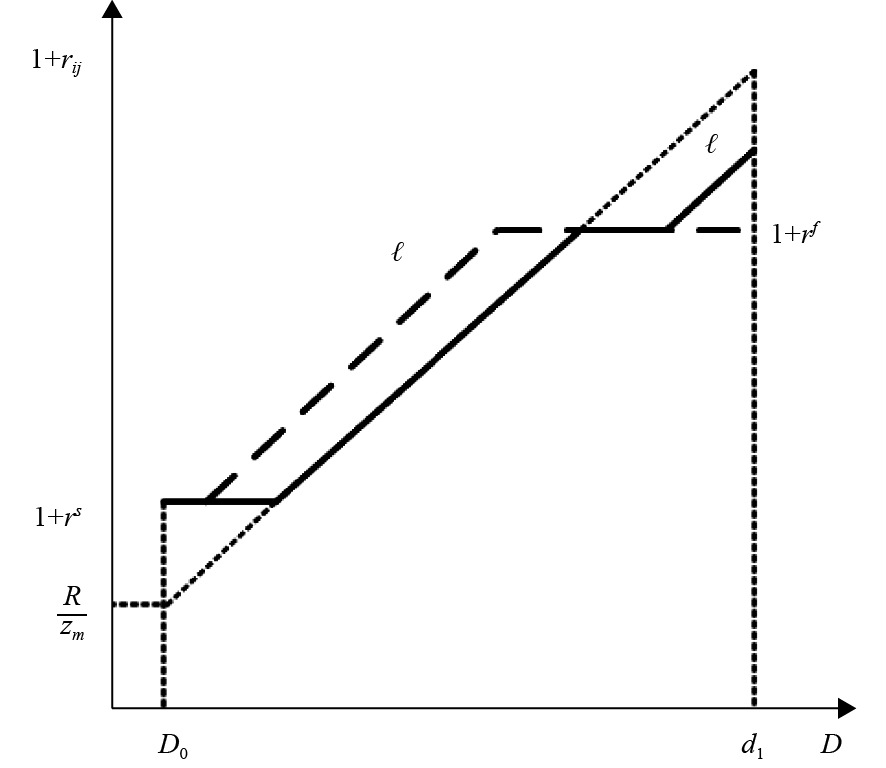图 4 抵押约束对同业拆借利率的影响

$\bar \rho {\rm{ = }}{{z_m^2}/{\phi \alpha }} \times$
$[{R/{{z_m}}} + {\phi /{z_m^2}} \times ({d_1} - 1 + \alpha ) - (1 + {r^f})]$
。因为是相机抉择，银行没有预期到，所以提高抵押率不会影响银行的资产配置（
$\alpha$
），成交利率曲线
$\ell$

$\bar \rho$
，以保证高流动性冲击发生时，拆借利率不会突破利率走廊上限。另外，与相机抉择策略不同，由于可以预期到抵押率提高，银行会增加其长期资产投资，导致成交利率曲线向左平移（
$\ell \to \ell '$
），但其分布区间不会超出走廊范围。

$R{\rm{ = }} 1.6$
${z_m} = 100$
$\phi = 300$
。另外，为了动态模拟市场利率的变化情况，我们将银行面临的冲击分解为两类：异质性冲击和系统性冲击，则可令
$\Phi = {D_{ik}} + D'(t) - 1 + \alpha$
，其中
$D'(t)$

$1 - \alpha$
）在上午已经决定，而流动性冲击（D）发生在中午，因此可将
$\Phi$

$\Phi > 0$

$\Phi < 0$

$\Phi (t)$
t表示第t天中午的流动性状况）来调控拆借利率。数值模拟过程为：首先，根据式（6）、式（16）和式（19），在同一时点求得成交利率的市场均值，即拆借利率；然后，根据拆借利率的不同波动形态，分析三种政策（公开市场操作、利率走廊与抵押政策）的调控效果。

$\Phi (t)$

$\eta = \theta \Phi (t) + (1 - \theta ) \Phi (t - 1)$
，其中
$\theta \in [0,1]$

$\theta$

$\eta$

$\theta$

$\theta = 1$
$\theta = 0.1$

$\theta = 1$
），恰当的公开市场操作能够实现市场利率的完全稳定。但当公开市场操作具有明显的滞后特点时（
$\theta = 0.1$
），市场利率的波动没有明显改善。图5（b进一步证实了这一结果。图5（b模拟了
$\theta$

$\theta$

$\theta$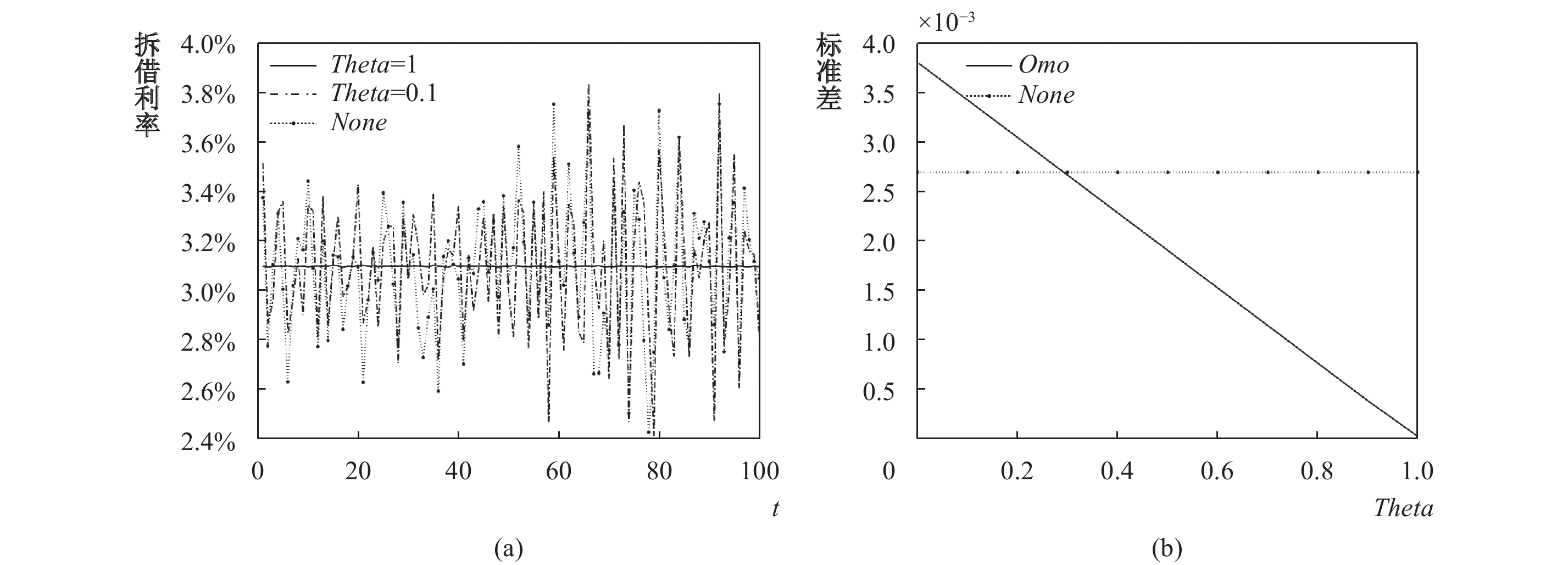图 5 公开市场操作与拆借利率波动

$high \to low$
），拆借利率降低，且其政策效果与央行的判断无关。这证实了上文的结论，即同幅度下调利率走廊上下限的政策属于扩张性货币政策。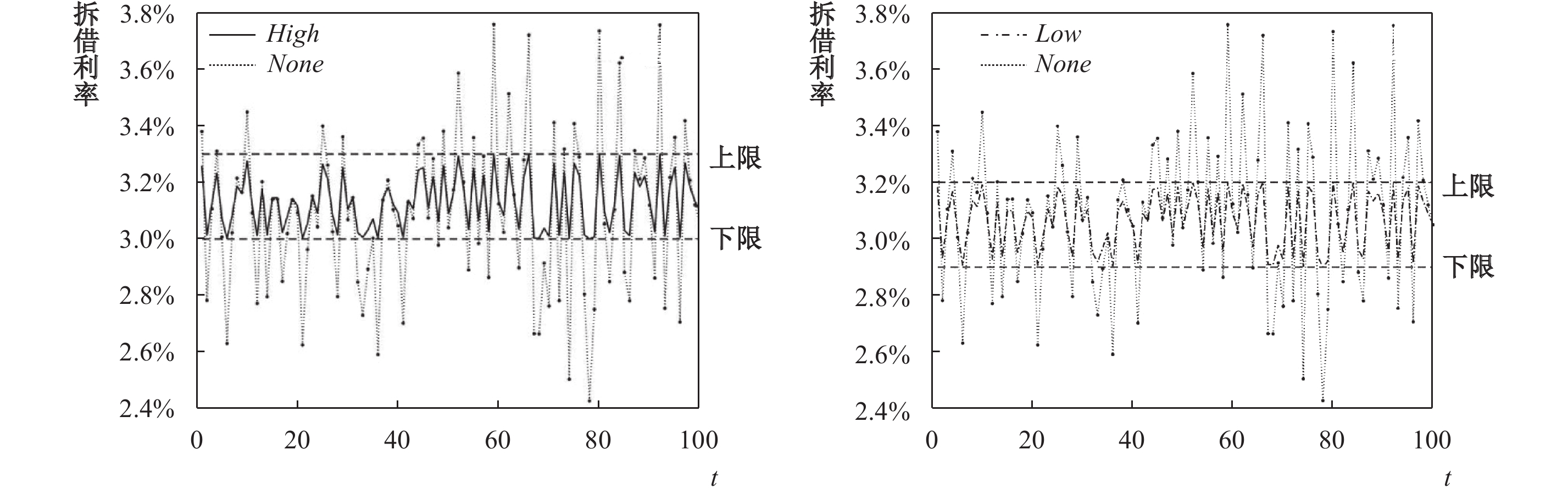图 6 利率走廊与拆借利率波动

$\rho {\rm{ = }} 0.5$
$\rho {\rm{ = }} 1$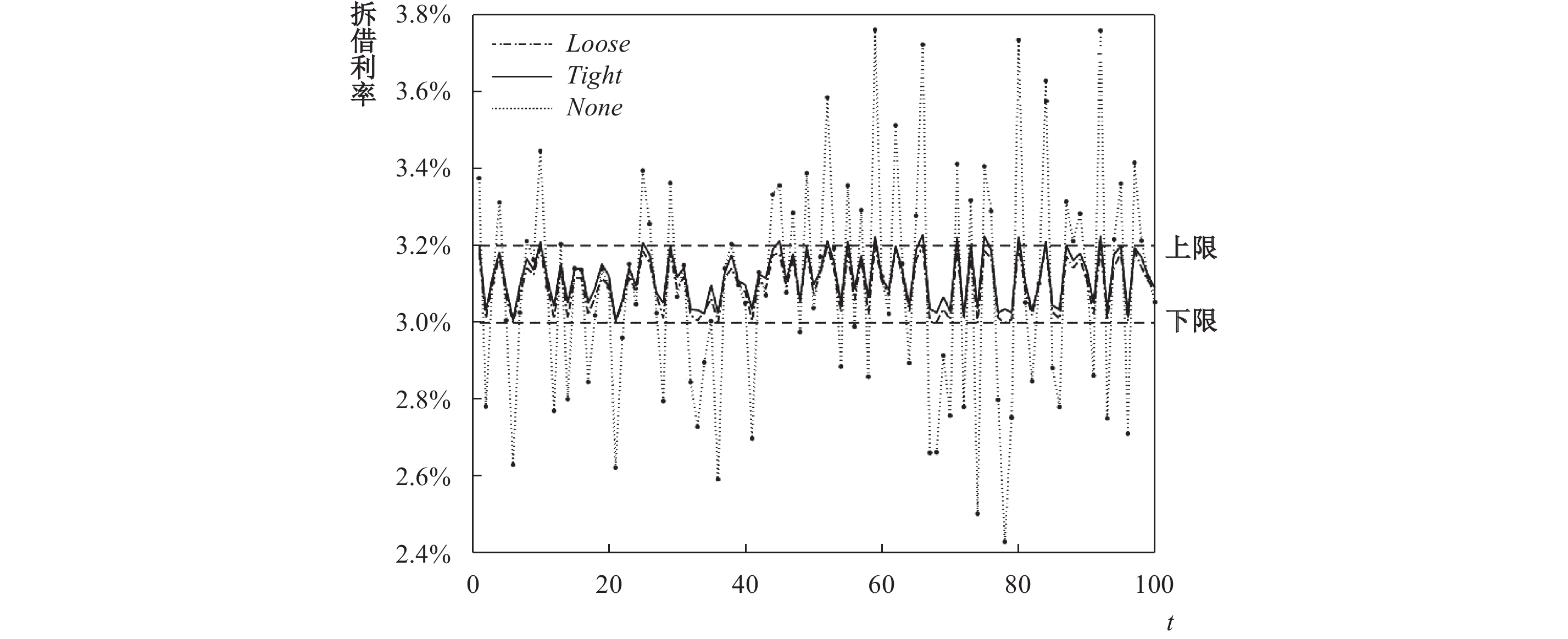图 7 抵押政策与拆借利率波动

* 感谢匿名审稿人及“中央财经大学宏观金融组”各位成员提出的宝贵意见与建议。文责自负。

① 一些文献已经证实，包括我国在内的世界上大多数国家的银行间市场是OTC市场（Ashcraft等, 2011；周荣芳，2015）。另外，中国人民银行制定的《同业拆借管理办法》第十五条规定，同业拆借交易以询价方式进行，自主谈判、逐笔成交；第十六条规定，同业拆借利率由交易双方自行商定。

  董小君. 从" 热钱”到" 钱荒”: 形势逆转的原因、影响及应对之策[J]. 经济学动态, 2013(7): 94–98.  马骏, 施康, 王红林, 等. 利率传导机制的动态研究[J]. 金融研究, 2016(1): 31–49.  牛慕鸿. 利率走廊运行机理再认识[J]. 中国金融, 2018(9): 25–27.  牛慕鸿, 张黎娜, 张翔. 利率走廊、利率稳定性和调控成本[J]. 金融研究, 2017(7): 16–28. DOI:10.3969/j.issn.1006-169X.2017.07.004  孙国峰, 蔡春春. 货币市场利率、流动性供求与中央银行流动性管理——对货币市场利率波动的新分析框架[J]. 经济研究, 2014(12): 33–44. DOI:10.3969/j.issn.2095-3151.2014.12.033  伍戈, 李斌. 货币数量、利率调控与政策转型[M]. 北京: 中国金融出版社, 2016.  杨光, 孙浦阳. 流动性过剩是否造成了" 钱荒”现象−基于异质性DSGE框架的分析[J]. 南开经济研究, 2015(5): 59–73.  张明. 银行间市场" 钱荒”的根源及政策含义[J]. 金融市场研究, 2013(7): 30–33.  张明, 郭子睿, 何帆. " 钱荒”为什么会发生? −上海银行间同业拆放利率的影响因素分析[J]. 国际金融研究, 2016(12): 84–93.  朱孟楠, 侯哲. 中国商业银行资金错配问题研究−基于" 钱荒”背景下的思考[J]. 国际金融研究, 2014(4): 62–69.  Ashcraft A, McAndrews J, Skeie D. Precautionary reserves and the interbank market[J]. Journal of Money, Credit and Banking, 2011, 43: 311–348. DOI:10.1111/jmcb.2011.43.issue-s2  Berentsen A, Monnet C. Monetary policy in a channel system[J]. Journal of Monetary Economics, 2008, 55(6): 1067–1080. DOI:10.1016/j.jmoneco.2008.07.002  Berentsen A, Marchesiani A, Waller C J. Channel systems: Why is there a positive spread? [R]. CESifo Working Paper No.3251, 2010. http://papers.ssrn.com/sol3/papers.cfm?abstract_id=1713686  Berentsen A, Marchesiani A, Waller C J. Floor systems for implementing monetary policy: Some unpleasant fiscal arithmetic[J]. Review of Economic Dynamics, 2014, 17(3): 523–542. DOI:10.1016/j.red.2013.09.001  Heider F, Hoerova M, Holthausen C. Liquidity hoarding and interbank market rates: The role of counterparty risk[J]. Journal of Financial Economics, 2015, 118(2): 336–354. DOI:10.1016/j.jfineco.2015.07.002  Whitesell W. Interest rate corridors and reserves[J]. Journal of Monetary Economics, 2006, 53(6): 1177–1195. DOI:10.1016/j.jmoneco.2005.03.013